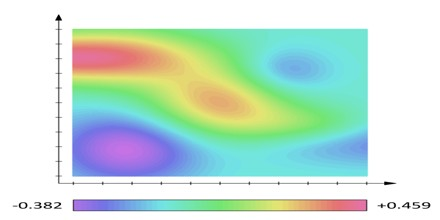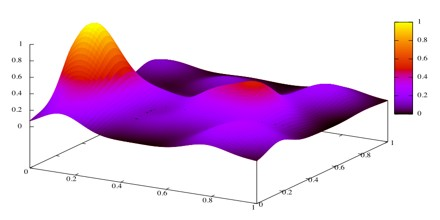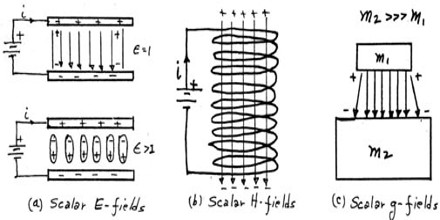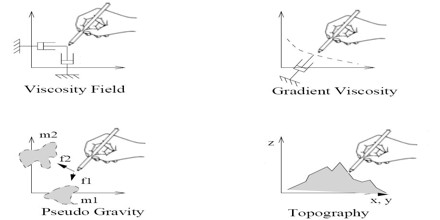Scalar Field

Definition

Scalar Field is a function that gives us a single value of some variable for every point in space. A scalar value is a single component that can assume one of a range of values. An example of this is temperature. In contrast, a vector value has more than one component. An example of a vector value is a direction, composed of x, y, and z components. The scalar may either be a mathematical number or a physical quantity.

Scalar Field data being visualized is not continuous, as the original scalar field is, but is composed of a set of discrete sampled values. The sample spacing may be regular, forming a grid, or the sample spacing might be irregular, with varied spacing between samples values.Thus, in ordinary three dimensional spaces the following are examples of scalar fields: sin xyz, cos z, x2 + y2 + z2. A linear field is one of the form ax + by + cz + d for some constants a, b, c and d.

In one dimension, an ordinary function is said to be differentiable at a point, if, when plotted against the variable, it looks like a straight line on a sufficiently small scale around that point.

A field defined in two dimensions is differentiable at the point (x’, y’) if its plot agains x and y looks like a plane on a sufficiently small scale around that point. The same statement is: the field is differentiable at (x’, y’) if it looks like a linear field and is approximated by one as closely as one wants at distances sufficiently close to (x’, y’). In this form differentiability is easy to visualize in any dimension.

Physically, a scalar field is additionally distinguished by having units of measurement associated with it. In this context, a scalar field should also be independent of the coordinate system used to describe the physical system—that is, any two observers using the same units must agree on the numerical value of a scalar field at any given point of physical space. Scalar fields are contrasted with other physical quantities such as vector fields, which associate a vector to every point of a region, as well as tensor fields and spinor fields. More subtly, scalar fields are often contrasted with pseudoscalar fields.Uses of Scalar Field

Scalar Fields are not located in a particular place, they permeate the universe. In a way they represent a smooth background, which may, however couple to other fields in physically interesting ways. To observe them is a challenge.

Scalar Fields are also supposed to cause the accelerated expansion of the universe (inflation), helping to solve the horizon problem and giving a hypothetical reason for the non-vanishing cosmological constant of cosmology. Massless (i.e. long-ranged) scalar fields in this context are known as inflatons. Massive (i.e. short-ranged) scalar fields are proposed, too, using for example Higgs-like fields.In mathematics and physics, a scalar field associates a scalar value to every point in a space. The scalar may either be a mathematical number or a physical quantity. Scalar fields are required to be coordinate-independent, meaning that any two observers using the same units will agree on the value of the scalar field at the same point in space (or spacetime). Examples used in physics include the temperature distribution throughout space, the pressure distribution in a fluid, and spin-zero quantum fields, such as the Higgs field. These fields are the subject of scalar field theory.

In scalar theories of gravitation scalar fields are used to describe the gravitational field.

Scalar-Tensor theories represent the gravitational interaction through both a tensor and a scalar. Such attempts are for example the Jordan theory as a generalization of the Kaluza–Klein theory and the Brans–Dicke theory.Difference between a Scalar Field and a Vector Field

• A scalar is a number, like 3 or 0.227. It has a bigness (3 is bigger than 0.227) but not a direction. Or not much of one; negative numbers go in the opposite direction from positive numbers, but that’s all. Numbers don’t go north or east or northeast. There is no such thing as a north 3 or an east 3.
• A vector is a special kind of complicated number that has a bigness and a direction. A vector like (1,0)(1,0) has bigness 1 and points east. The vector (0,1)(0,1) has the same bigness but points north. The vector (0,2)(0,2) also points north, but is twice as big as (0,2)(0,2). The vector (1,1)(1,1) points northeast, and has a bigness of 2–√2, so it’s bigger than (0,1)(0,1) but smaller than (0,2)(0,2).
• A scalar field means we take some space, say a plane, and measure some scalar value at each point.
• A vector field means we take some space, say a plane, and measure some vector value at each point.
• A scalar field is simply a single function of, say n variables.
• A vector is a set of functions of n variables.